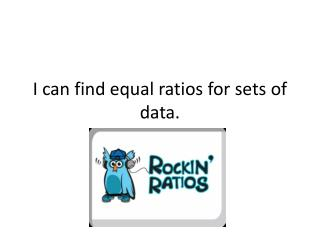Download PresentationI can find equal ratios for sets of data.

# I can find equal ratios for sets of data. - PowerPoint PPT PresentationDownload Presentation## I can find equal ratios for sets of data.

- - - - - - - - - - - - - - - - - - - - - - - - - - - E N D - - - - - - - - - - - - - - - - - - - - - - - - - - -
##### Presentation Transcript

1. I can find equal ratios for sets of data.

2. Think about it…. What fraction of objects are squares? What is the ratio of triangles to squares?

3. In your notes…. Comparison of two numbers by division. Written 3 ways 3 to 4 3 : 4 3/4 Ratio

4. Write the ratio in three different ways • In a certain district, schools average 182 pupils for every 10 teachers. • In a recent year, 41 out of 250 people liked Justin Beiber. • 284 out of 1,000 people are 15-17 years old. • 7 out of every 10 people have visited California.

5. 7th grade students asked whether they like pizza or hamburgers better. 1. In Mrs. McClain’s room what is the ratio of students who prefer hamburgers to pizza? 2. Combine the totals for all three rooms. What is the ratio of the number of students who prefer pizza to the number of students who prefer hamburgers?

6. Write each ratio in simplest form (reduce just like fractions) • 2:6 • 3:21 • 16 to 20 • 3/30 • 49: 14

7. Express each ratio as a decimal(Divide the first number by the 2nd number) • 7:8 • 8/3 • 12:25 • 1:2 0.9 2.7 0.5 0.5

8. Write two equivalent ratios • 26:54 • 212:100 • 18:36 • 1:2 • 9:10

9. Mr. Burns class has a ratio of 13 male students to 14 female students. The entire school population has 273 male students and 294 female students. Is this the same as the ratio in Mr. Burn’s class?

10. An average adult male gorilla living in the wild weighs about 350 lb. In zoos, an adult male gorilla can weigh about 750 lb. Write a ratio in simplest form to compare the weights of the gorillas in the two different habitats. 7/15

11. Challenge • A bag contains red, blue, and yellow marbles. The ratio of red marbles to blue marbles is 1:4. The ratio of blue marbles to yellow marbles is 2:5. What is the ratio of red marbles to yellow marbles? 1:10

12. Wrap up • What is a ratio? • Explain the different ways you can express ratios.

13. I Can Find Unit Rates

14. In your notes…. A ratio that compares two quantities measured in different units. \$10.00/3 pizzas Rate Unit Rate